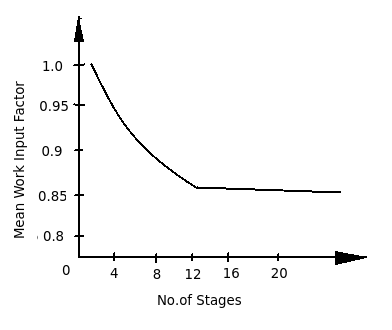# Effect of increasing number of stages on mean work input factor in axial flow compressor

Q.  What is the effect of increasing number of stages in axial flow compressor on the mean work input factor (Ψw)?
- Published on 16 Sep 15

a. Mean work input factor (Ψw) decreases
b. Mean work input factor (Ψw) increases
c. Mean work input factor (Ψw) remains constant
d. Unpredictable

ANSWER: Mean work input factor (Ψw) decreases

#### Discussion

• Pankaj   -Posted on 16 Oct 15
- The ratio of actual work absorbed by the axial flow compressor to the theoretical work is called as workdone factor or work input factor, (Ψw).

- The overall capacity of work in a stage reduces die to the variation in work absorbing capacities at the central region of the flow and near the hub and casing.

- Therefore, mean work input factor of an axial flow compressor decreases with increase in number of stages as shown in diagram.## ➨ Post your comment / Share knowledgeEnter the code shown above:

(Note: If you cannot read the numbers in the above image, reload the page to generate a new one.)